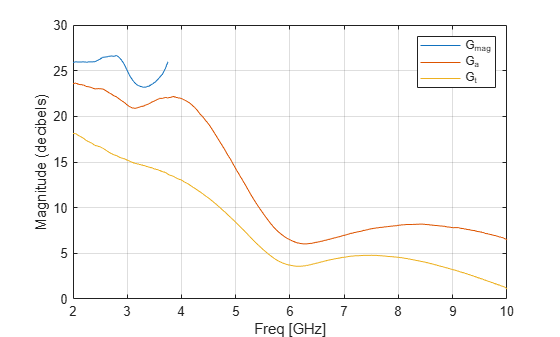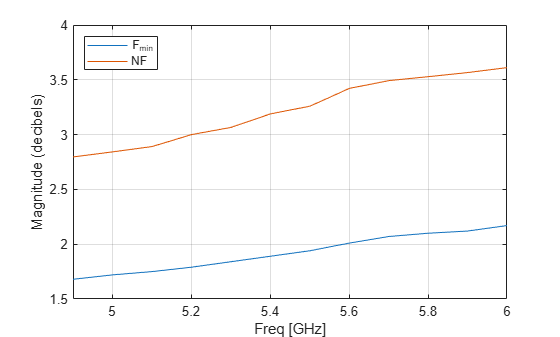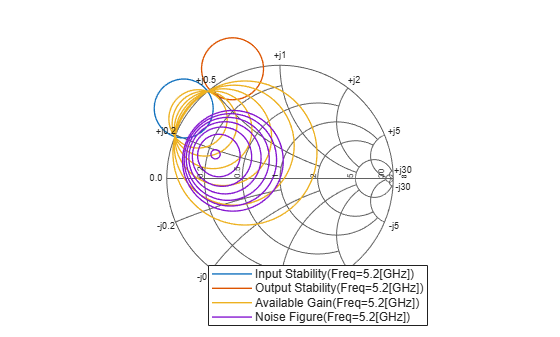Documentation

## Designing Matching Networks (Part 1: Networks with an LNA and Lumped Elements)

This example shows how to verify the design of input and output matching networks for a Low Noise Amplifier (LNA) by plotting its gain and noise.

In wireless communications, receivers need to be able to detect and amplify incoming low-power signals without adding much noise. Therefore, a LNA is often used as the first stage of these receivers. To design an LNA, this example uses the available gain design technique, which involves selecting an appropriate matching network that provides a suitable compromise between gain and noise.

### LNA Design Specifications

The LNA design specifications are as follows:

• Frequency range: 5.10 - 5.30 GHz

• Noise Figure <= 2.2 dB

• Transducer Gain > 11 dB

• Operating between 50-ohm terminations

### Create an `rfckt.amplifier` Object and Examine the Amplifier Power Gains and Noise Figure

Create an `rfckt.amplifier` object to represent the amplifier that is specified in the file, 'samplelna1.s2p'. `analyze` the amplifier in the frequency range from 2 GHz to 10 GHz. `plot` the transducer power gain (`Gt`), the available power gain (`Ga`) and the maximum available power gain (`Gmag`).

```unmatched_amp = read(rfckt.amplifier, 'samplelna1.s2p'); analyze(unmatched_amp, 2e9:50e6:10e9); figure plot(unmatched_amp,'Gmag','Ga','Gt','dB') ```This example shows how to design the input and output matching networks at 5.2 GHz, so examine the power gains at this frequency. Without the input and output matching networks, the transducer power gain at 5.2 GHz is about 7.2 dB; it is below the gain requirement of 11 dB in the specification and less than the available power gain. This amplifier is also potentially unstable at 5.2 GHz, because the maximum available gain does not exist at 5.2 GHz.

Plot the measured minimum noise figure (`Fmin`) and the noise figure (`NF`) calculated when there is no input matching network. Specify an-axis range of 4.9 GHz to 6 GHz, where the minimum noise figure is measured.

```plot(unmatched_amp,'Fmin','NF','dB') axis([4.9 6 1.5 4]) legend('Location','NorthWest') ```When there is no input matching network, the noise figure between 5.10 and 5.30 GHz is above the noise figure requirement of 2.2 dB in the specification.

### Plot Gain, Noise Figure and Stability Circles

Both the available gain and the noise figure are functions of the source reflection coefficient, GammaS. To select an appropriate GammaS that provides a suitable compromise between gain and noise, use the `circle` method of the `rfckt.amplifier` object to place the constant available gain and the constant noise figure circles on the Smith chart. As mentioned earlier, the amplifier is potentially unstable at 5.2 GHz. So, the following `circle` command also places the input and output stability circles on the Smith chart.

```fc = 5.2e9; hsm = smithplot; circle(unmatched_amp,fc,'Stab','In','Stab','Out','Ga',10:2:20, ... 'NF',1.8:0.2:3,hsm); legend('Location','SouthEast') ```Enable the data cursor and click on the constant available gain circle. The data tip displays the following data:

• Available power gain (`Ga`)

• Noise figure (`NF`)

• Source reflection coefficient (`GammaS`)

• Output reflection coefficient (`GammaOut`)

• Normalized source impedance (`ZS`)

`Ga`, `NF`, `GammaOut` and `ZS` are all functions of the source reflection coefficient, `GammaS`. `GammaS` is the complex number that corresponds to the location of the data cursor. A star ('*') and a circle-in-dashed-line will also appear on the Smith chart. The star represents the matching load reflection coefficient (`GammaL`) that is the complex conjugate of `GammaOut`. The gain is maximized when `GammaL` is the complex conjugate of `GammaOut`. The circle-in-dashed-line represents the trajectory of the matching `GammaL` when the data cursor moves on a constant available gain or noise figure circle.Because both the `S11` and `S22` parameters of the amplifier are less than unity in magnitude, both the input and output stable region contain the center of the Smith chart. In order to make the amplifier stable, `GammaS` must be in the input stable region and the matching `GammaL` must be in the output stable region. The output stable region is shaded in the above figure. However, when a `GammaS` that gives a suitable compromise between gain and noise is found, the matching `GammaL` always falls outside the output stable region. So we must stabilize the amplifier first.

### Stabilize the Amplifier

One way to stabilize an amplifier is to cascade a shunt resistor at the output of the amplifier. However, this approach will also reduce gain and add noise. At the end of the example, we will verify that the overall gain and noise still meet the requirement.

To find the maximum shunt resistor value that makes the amplifier unconditionally stable, use the `fzero` function to find the resistor value that makes stability `MU` equal to 1. The `fzero` function always tries to achieve a value of zero for the objective function, so the objective function should return `MU-1`.

```type('lna_match_stabilization_helper.m') ```
```function mu_minus_1 = lna_match_stabilization_helper(propval, fc, ckt, element, propname) %LNA_MATCH_STABILIZATION_HELPER Return Stability MU-1. % MU_MINUS_1 = LNA_MATCH_STABILIZATION_HELPER(PROPVALUE, FC, CKT, % ELEMENT, PROPNAME) returns stability parameter MU-1 of a circuit, CKT % when the property called PROPNAME of an element, ELEMENT is set to % PROPVAL. % % LNA_MATCH_STABILIZATION_HELPER is a helper function of RF % Toolbox demo: Designing Matching Networks (Part 1: Networks with an LNA % and Lumped Elements). % Copyright 2007-2008 The MathWorks, Inc. set(element, propname, propval) analyze(ckt, fc); mu_minus_1 = stabilitymu(ckt.AnalyzedResult.S_Parameters) - 1; ```

Compute the parameters for the objective function and pass the objective function to `fzero` to get the maximum shunt resistor value.

```stab_amp = rfckt.cascade('ckts', {unmatched_amp, rfckt.shuntrlc}); R1 = fzero(@(R1) lna_match_stabilization_helper(R1,fc,stab_amp,stab_amp.Ckts{2},'R'),[1 1e5]) ```
```R1 = 118.6213 ```

### Find GammaS and GammaL

Cascade a 118-ohm resistor at the output of the amplifier and analyze the cascade. Place the new constant available gain and the constant noise figure circles on the Smith chart.

```shunt_r = rfckt.shuntrlc('R',118); stab_amp = rfckt.cascade('ckts',{unmatched_amp,shunt_r}); analyze(stab_amp,fc); hsm = smithplot; circle(stab_amp,fc,'Ga',10:17,'NF',1.80:0.2:3,hsm) legend('Location','SouthEast') ```Use the data cursor to locate a `GammaS` where there is a suitable compromise between gain and noise. The example selects a `GammaS` that gives gain of 14 dB and noise figure of 1.84 dB. Compute the matching `GammaL`, which is the complex conjugate of `GammaOut` on the data tip.```GammaS = 0.67*exp(1j*153.6*pi/180) ```
```GammaS = -0.6001 + 0.2979i ```

Compute the normalized source impedance.

```Zs = gamma2z(GammaS,1) ```
```Zs = 0.2080 + 0.2249i ```

Compute the matching `GammaL` that is equal to the complex conjugate of `GammaOut`.

```GammaL = 0.7363*exp(1j*120.1*pi/180) ```
```GammaL = -0.3693 + 0.6370i ```

```Zl = gamma2z(GammaL,1) ```
```Zl = 0.2008 + 0.5586i ```

### Design the Input Matching Network Using GammaS

In this example, the lumped LC elements are used to build the input and output matching networks as follows:The input matching network consists of one shunt capacitor, Cin, and one series inductor, Lin. Use the Smith chart and the data cursor to find component values. To do this, start by plotting the constant conductance circle that crosses the center of the Smith chart and the constant resistance circle that crosses `GammaS`.

```hsm = smithplot; circle(stab_amp,fc,'G',1,'R',real(Zs),hsm); hsm.GridType = 'YZ'; hold all plot(GammaS,'k.','MarkerSize',16) text(real(GammaS)+0.05,imag(GammaS)-0.05,'\Gamma_{S}','FontSize', 12, ... 'FontUnits','normalized') plot(0,0,'k.','MarkerSize',16) hold off ```Then, find the intersection points of the constant conductance and the constant resistance circle. Based on the circuit diagram above, the intersection point in the lower half of the Smith chart should be used. Mark it as point A.```GammaA = 0.6983*exp(1j*(-134.3)*pi/180); Za = gamma2z(GammaA,1); Ya = 1/Za; ```

Determine the value of `Cin` from the difference in susceptance from the center of the Smith chart to point A. Namely,

``

where 50 is the reference impedance.

```Cin = imag(Ya)/50/2/pi/fc ```
```Cin = 1.1945e-12 ```

Determine the value of `Lin` from the difference in reactance from point A to `GammaS`. Namely,

``
```Lin = (imag(Zs) - imag(Za))*50/2/pi/fc ```
```Lin = 9.6522e-10 ```

### Design the Output Matching Network Using GammaL

Use the approach described in the previous section on designing the input matching network to design the output matching network and get the values of `Cout` and `Lout`.

```GammaB = 0.7055*exp(1j*(-134.9)*pi/180); Zb = gamma2z(GammaB, 1); Yb = 1/Zb; Cout = imag(Yb)/50/2/pi/fc ```
```Cout = 1.2194e-12 ```
```Lout = (imag(Zl) - imag(Zb))*50/2/pi/fc ```
```Lout = 1.4682e-09 ```

### Verify the Design

Create the input and output matching networks. Cascade the input matching network, the amplifier, the shunt resistor and the output matching network to build the LNA.

```input_match = rfckt.cascade('Ckts', ... {rfckt.shuntrlc('C',Cin),rfckt.seriesrlc('L',Lin)}); output_match = rfckt.cascade('Ckts', ... {rfckt.seriesrlc('L',Lout),rfckt.shuntrlc('C',Cout)}); LNA = rfckt.cascade('ckts', ... {input_match,unmatched_amp,shunt_r,output_match}); ```

Analyze the LNA around the design frequency range and plot the available and transducer power gain. The available and transducer power gain at 5.2 GHz are both 14 dB as the design intended. The transducer power gain is above 11 dB in the design frequency range, which meets the requirement in the specification.

```analyze(LNA,5.05e9:10e6:5.35e9); plot(LNA,'Ga','Gt','dB'); ```Plot the noise figure around the design frequency range. The noise figure is below 2.2 dB in the design frequency range, which also meets the requirement in the specification. The noise figure of the LNA at 5.2 GHz is about 0.1 dB above that of the amplifier (1.84 dB), which demonstrates added noise by the shunt resistor.

```plot(LNA,'NF','dB') ```The available gain design method is often used in LNA matching. There are other design methods for other devices. In the second part of the example -- Designing Matching Networks (Part 2: Single Stub Transmission Lines), a simultaneous conjugate matching example is presented.